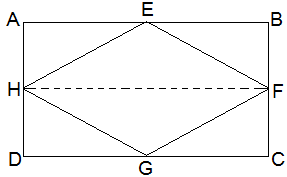Guru

# If E, F, G and H are respectively the mid-points of the sides of a parallelogram ABCD, show that ar (EFGH) = 1/2 ar(ABCD). Q.2

• 0

I want to know the best answer of the question from Areas of Parallelograms and Triangles chapter of class 9th ncert math. The question from exercise 9.2of math. Give me the easy way for solving this question of 2 If E, F, G and H are respectively the mid-points of the sides of a parallelogram ABCD, show that ar (EFGH) = 1/2 ar(ABCD)

Share

1.Given,

E, F, G and H are the mid-points of the sides of a parallelogram ABCD respectively.

To Prove,

ar (EFGH) = ½ ar(ABCD)

Construction,

H and F are joined.

Proof,

AD || BC and AD = BC (Opposite sides of a parallelogram)

⇒ ½ AD = ½ BC

Also,

AH || BF and and DH || CF

⇒ AH = BF and DH = CF (H and F are mid points)

∴, ABFH and HFCD are parallelograms.

Now,

We know that, ΔEFH and parallelogram ABFH, both lie on the same FH the common base and in-between the same parallel lines AB and HF.

∴ area of EFH = ½ area of ABFH — (i)

And, area of GHF = ½ area of HFCD — (ii)